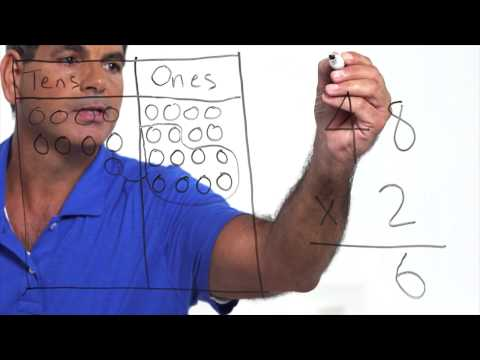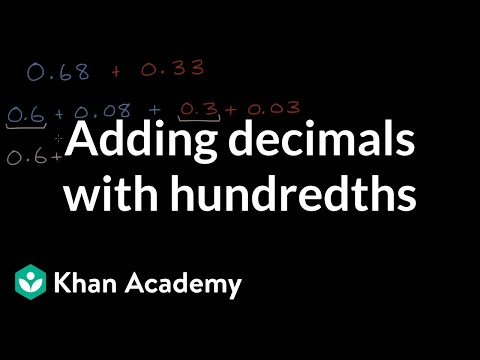Video

# Intro to multiplying decimals (Full video)

Description: Sal introduces multiplying decimals with problems like 9 × 0.6. Created by Sal Khan. Let's see if we can multiply 9 times 0.6. Or another way to write it, we want to calculate 9 times 0.6. We know that if we start with 6, which we could write as 6.0, and if you were to divide it by 10, dividing by 10 is equivalent to moving the decimal place one place to the left.

### Other videos you might be interested in### Math Studio Talk: Instruction for 5.NBT (Full video)

#### EngageNY